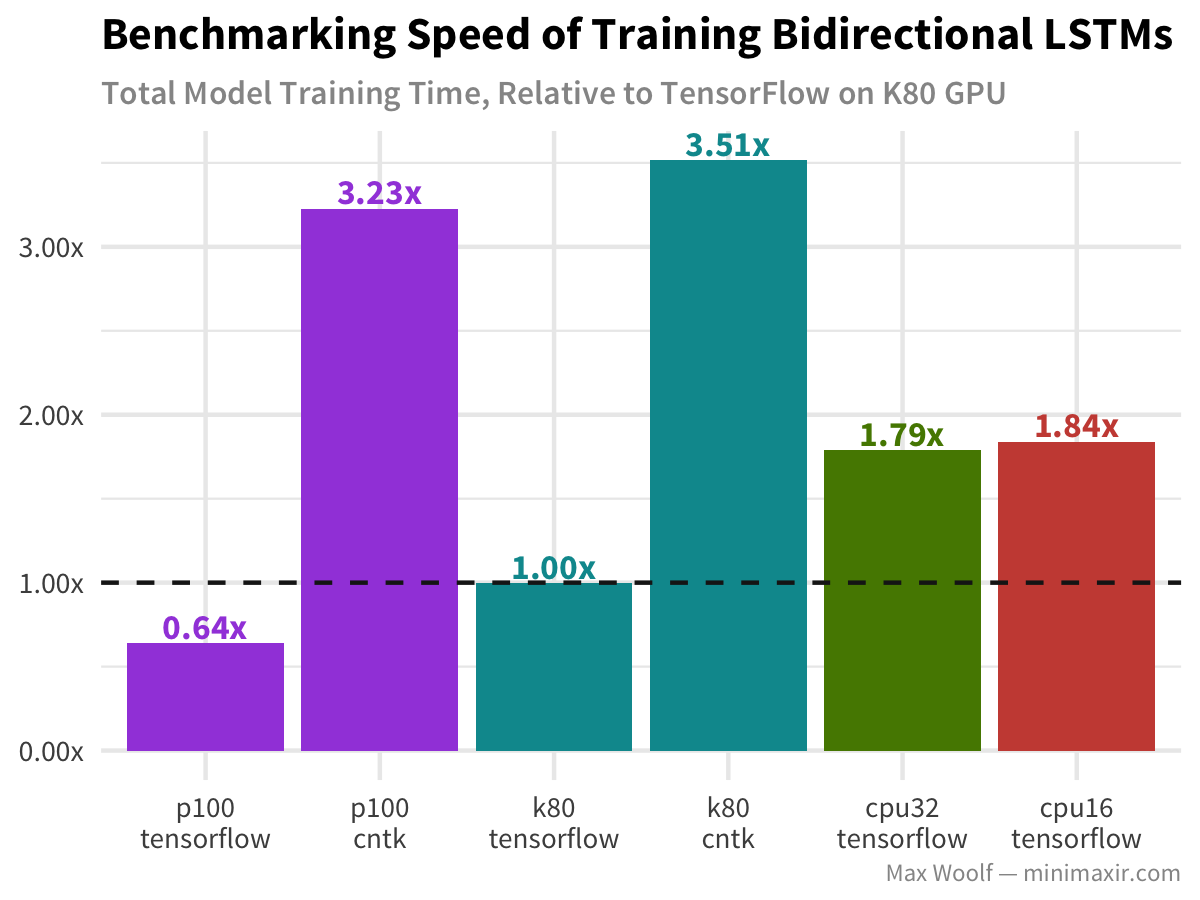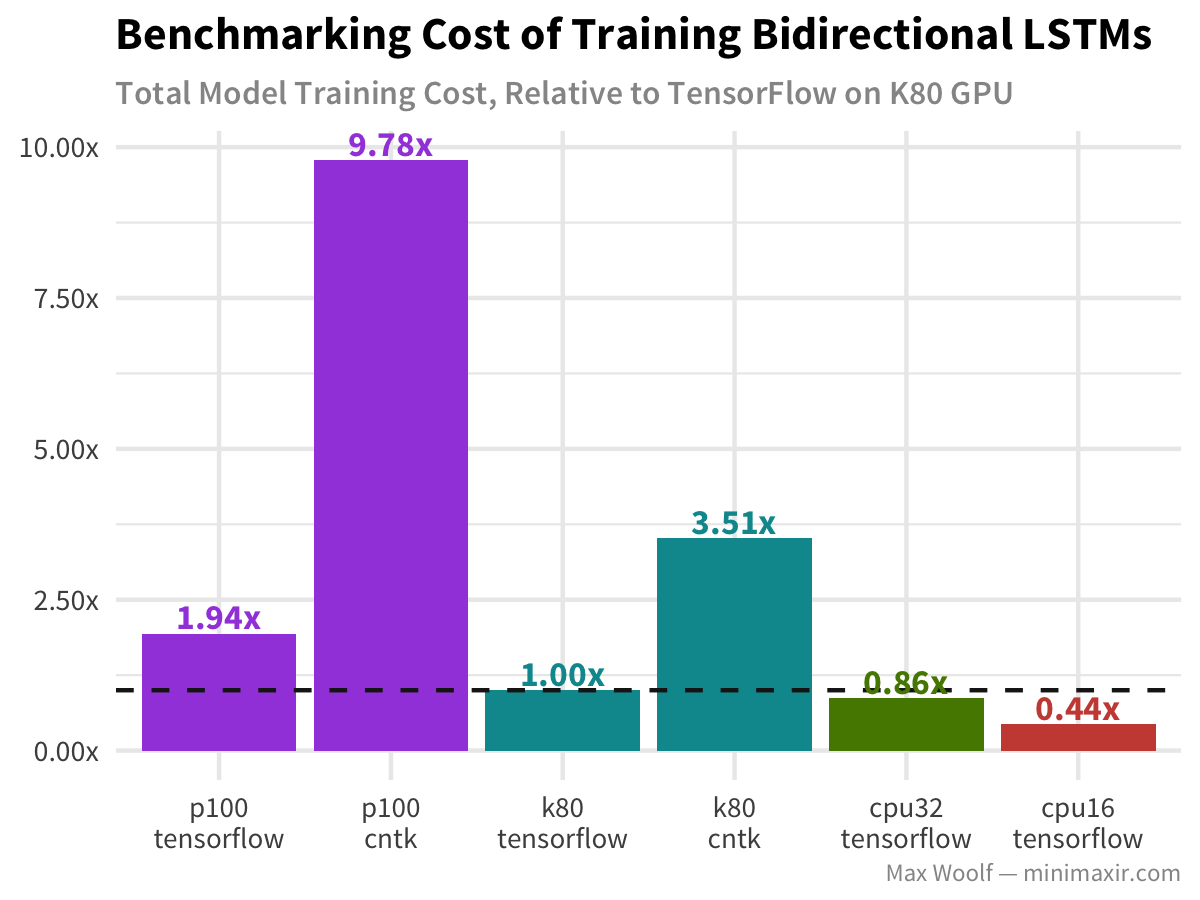This R Notebook is the complement to my blog post Benchmarking Modern GPUs for Maximum Cloud Cost Efficiency in Deep Learning.

This notebook is licensed under the MIT License. If you use the code or data visualization designs contained within this notebook, it would be greatly appreciated if proper attribution is given back to this notebook and/or myself. Thanks! :)

# 1 Setup

``````library(scales)
library(tidyverse)``````
``````[30m── [1mAttaching packages[22m ────────────────────────────── tidyverse 1.2.1 ──[39m
[30m[32m✔[30m [34mggplot2[30m 2.2.1.[31m9000[30m     [32m✔[30m [34mpurrr  [30m 0.2.4
[32m✔[30m [34mtibble [30m 1.3.4          [32m✔[30m [34mdplyr  [30m 0.7.4
[32m✔[30m [34mtidyr  [30m 0.7.2          [32m✔[30m [34mstringr[30m 1.2.0
[32m✔[30m [34mreadr  [30m 1.1.1          [32m✔[30m [34mforcats[30m 0.2.0     [39m
[30m── [1mConflicts[22m ───────────────────────────────── tidyverse_conflicts() ──
[31m✖[30m [34mdplyr[30m::[32mfilter()[30m     masks [34mstats[30m::filter()
[31m✖[30m [34mdplyr[30m::[32mlag()[30m        masks [34mstats[30m::lag()[39m``````
``````library(RColorBrewer)
sessionInfo()``````
``````R version 3.4.2 (2017-09-28)
Platform: x86_64-apple-darwin15.6.0 (64-bit)
Running under: macOS High Sierra 10.13.1

Matrix products: default
BLAS: /System/Library/Frameworks/Accelerate.framework/Versions/A/Frameworks/vecLib.framework/Versions/A/libBLAS.dylib
LAPACK: /Library/Frameworks/R.framework/Versions/3.4/Resources/lib/libRlapack.dylib

locale:
 en_US.UTF-8/en_US.UTF-8/en_US.UTF-8/C/en_US.UTF-8/en_US.UTF-8

attached base packages:
 stats     graphics  grDevices utils     datasets  methods
 base

other attached packages:
 RColorBrewer_1.1-2 forcats_0.2.0      stringr_1.2.0
 dplyr_0.7.4        purrr_0.2.4        readr_1.1.1
 tidyr_0.7.2        tibble_1.3.4       ggplot2_2.2.1.9000
 tidyverse_1.2.1    scales_0.5.0

loaded via a namespace (and not attached):
 Rcpp_0.12.14     cellranger_1.1.0 compiler_3.4.2
 plyr_1.8.4       bindr_0.1        tools_3.4.2
 jsonlite_1.5     lubridate_1.7.1  nlme_3.1-131
 gtable_0.2.0     lattice_0.20-35  pkgconfig_2.0.1
 rlang_0.1.4      psych_1.7.8      cli_1.0.0
 rstudioapi_0.7   yaml_2.1.14      parallel_3.4.2
 haven_1.1.0      bindrcpp_0.2     xml2_1.1.1
 httr_1.3.1       knitr_1.17       hms_0.4.0
 grid_3.4.2       glue_1.2.0       R6_2.2.2
 readxl_1.0.0     foreign_0.8-69   modelr_0.1.1
 reshape2_1.4.2   magrittr_1.5     rvest_0.3.2
 assertthat_0.2.0 mnormt_1.5-5     colorspace_1.3-2
 stringi_1.1.6    lazyeval_0.2.1   munsell_0.4.3
 broom_0.4.3      crayon_1.3.4    ``````

Set ggplot2 theme.

``````theme_set(theme_minimal(base_size=9, base_family="Source Sans Pro") +
theme(plot.title = element_text(size=11, family="Source Sans Pro Bold"),
axis.title.x = element_blank(),
axis.title.y = element_blank(),
plot.subtitle = element_text(family="Source Sans Pro Semibold", color="#969696"),
plot.caption = element_text(size=6, color="#969696"),
axis.text.x = element_text(size = 7),
legend.position="none"))``````
``````relative <- function(x) {
lab <- paste0(sprintf("%.2f", x), 'x')
}``````

Set known price rates from Google Compute Engine Pricing.

``````standard_cost_hr <- 0.0475
k80_cost_hr <- 0.450
p100_cost_hr <- 1.460
cpu16_cost_hr <- 0.120``````

Derive the remaining rates, in seconds.

``````k80_cost_s <- (k80_cost_hr + standard_cost_hr) / 3600
p100_cost_s <- (p100_cost_hr + standard_cost_hr) / 3600
cpu16_cost_s <- cpu16_cost_hr / 3600
cpu32_cost_s <- cpu16_cost_s * 2
# works like a Python dict
costs <- c(k80=k80_cost_s, p100=p100_cost_s, cpu16=cpu16_cost_s, cpu32=cpu32_cost_s)``````

# 2 Analysis

Create a helper function to return the results for all permutations of a given test file name.

``````# frameworks <- c('tensorflow','cntk')
# platforms <- c("p100", "k80", "cpu32", "cpu16")
labels <- c('p100-tensorflow','p100-cntk', 'k80-tensorflow','k80-cntk', 'cpu32-tensorflow','cpu16-tensorflow')
process_test_data <- function(file_name) {
base_label <- 'k80-tensorflow'
label_split <- str_split(base_label, "-")[]
label_str <- paste(label_split, label_split, sep="\n")
results <- read_csv(sprintf("../logs/%s/%s_%s.csv", label_split, file_name, label_split), col_types = cols()) %>%
mutate(platform = label_split, framework = label_split) %>%
group_by(platform, framework) %>%
summarize(total_time = sum(elapsed),
total_cost = total_time * costs[label_split]) %>%
mutate(label = label_str)

base_total_time <- results %>% pull(total_time)
base_total_cost <- results %>% pull(total_cost)

labels_tf <- labels[!(labels %in% base_label)]

for(i in 1:length(labels_tf)) {
label = labels_tf[i]
label_split <- str_split(label, "-")[]
label_str <- paste(label_split, label_split, sep="\n")
temp_df <- read_csv(sprintf("../logs/%s/%s_%s.csv", label_split, file_name, label_split), col_types = cols()) %>%
mutate(platform = label_split,
framework = label_split) %>%
group_by(platform, framework) %>%
summarize(total_time = sum(elapsed),
total_cost = total_time * costs[label_split]) %>%
ungroup() %>%
mutate(label = label_str)

results <- results %>% bind_rows(temp_df)

}
# Normalize
results_final <- results %>%
as_tibble() %>%
mutate(total_time_norm = total_time / base_total_time,
total_cost_norm = total_cost / base_total_cost,
label = as_factor(label)
)

return(results_final)

}
process_test_data('cifar10_cnn')``````
``Unequal factor levels: coercing to characterbinding character and factor vector, coercing into character vectorbinding character and factor vector, coercing into character vectorbinding character and factor vector, coercing into character vectorbinding character and factor vector, coercing into character vector``

## 2.1 IMDB Bidirectional LSTM

``df_imdb_lstm <- process_test_data("imdb_bidirectional_lstm")``
``Unequal factor levels: coercing to characterbinding character and factor vector, coercing into character vectorbinding character and factor vector, coercing into character vectorbinding character and factor vector, coercing into character vectorbinding character and factor vector, coercing into character vector``
``df_imdb_lstm``
``````plot <- ggplot(df_imdb_lstm, aes(x=fct_rev(label), y=total_time_norm, fill=platform)) +
geom_bar(stat="identity") +
geom_hline(yintercept = 1, linetype="dashed", color="#1a1a1a") +
geom_text(aes(label = relative(total_time_norm), color=platform), vjust=-0.2, family="Source Sans Pro Bold", size=3) +

scale_y_continuous(labels = relative) +
scale_fill_hue(l=50) +
scale_color_hue(l=50) +
labs(title = "Benchmarking Speed of Training Bidirectional LSTMs",
x = "Platform",
subtitle = "Total Model Training Time, Relative to TensorFlow on K80 GPU",
caption = "Max Woolf — minimaxir.com")
ggsave("dl-cpu-gpu-1.png", plot, width=4, height=3)````````````plot <- ggplot(df_imdb_lstm, aes(x=fct_rev(label), y=total_cost_norm, fill=platform)) +
geom_bar(stat="identity") +
geom_hline(yintercept = 1, linetype="dashed", color="#1a1a1a") +
geom_text(aes(label = relative(total_cost_norm), color=platform), vjust=-0.2, family="Source Sans Pro Bold", size=3) +
scale_y_continuous(labels = relative) +
scale_fill_hue(l=50) +
scale_color_hue(l=50) +
labs(title = "Benchmarking Cost of Training Bidirectional LSTMs",
x = "Platform",
subtitle = "Total Model Training Cost, Relative to TensorFlow on K80 GPU",
caption = "Max Woolf — minimaxir.com")
ggsave("dl-cpu-gpu-2.png", plot, width=4, height=3)``````## 2.2 IMDB Fasttext

``df_imdb_fasttext <- process_test_data("imdb_fasttext")``
``Unequal factor levels: coercing to characterbinding character and factor vector, coercing into character vectorbinding character and factor vector, coercing into character vectorbinding character and factor vector, coercing into character vectorbinding character and factor vector, coercing into character vector``
``df_imdb_fasttext``
``````plot <- ggplot(df_imdb_fasttext, aes(x=fct_rev(label), y=total_time_norm, fill=platform)) +
geom_bar(stat="identity") +
geom_hline(yintercept = 1, linetype="dashed", color="#1a1a1a") +
geom_text(aes(label = relative(total_time_norm), color=platform), vjust=-0.2, family="Source Sans Pro Bold", size=3) +

scale_y_continuous(labels = relative) +
scale_fill_hue(l=50) +
scale_color_hue(l=50) +
labs(title = "Benchmarking Speed of Training fasttext",
x = "Platform",
subtitle = "Total Model Training Time, Relative to TensorFlow on K80 GPU",
caption = "Max Woolf — minimaxir.com")
ggsave("dl-cpu-gpu-3.png", plot, width=4, height=3)``````